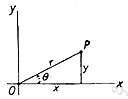# abscissa

(redirected from abscisse)
Also found in: Thesaurus, Medical, Encyclopedia.

## ab·scis·sa

(ăb-sĭs′ə)
n. pl. ab·scis·sas or ab·scis·sae (-sĭs′ē)
Symbol x The coordinate representing the position of a point along a line perpendicular to the y-axis in a plane Cartesian coordinate system.

[New Latin (līnea) abscissa, (line) cut off, from Latin abscissa, feminine past participle of abscindere, to abscise; see abscission.]

## abscissa

(æbˈsɪsə)
n, pl -scissas or -scissae (-ˈsɪsiː)
(Mathematics) the horizontal or x-coordinate of a point in a two-dimensional system of Cartesian coordinates. It is the distance from the y-axis measured parallel to the x-axis. Also : absciss or abscisse Compare ordinate
[C17: New Latin, originally linea abscissa a cut-off line]

## ab•scis•sa

(æbˈsɪs ə)

n., pl. -scis•sas, -scis•sae (-ˈsɪs i)
(in plane Cartesian coordinates) the x-coordinate of a point: its distance from the y-axis measured parallel to the x-axis. Compare ordinate.
[1690–1700; < Latin, feminine of abscissus, past participle of abscindere to cut off =ab- ab- + scindere to divide, tear]

## ab·scis·sa

(ăb-sĭs′ə)
The distance of a point from the y-axis on a graph in the Cartesian coordinate system. It is measured parallel to the x-axis. For example, a point having coordinates (2,3) has 2 as its abscissa. Compare ordinate.
ordinate, abscissa - The ordinate or Y axis is vertical; the abscissa or X axis is horizontal.
 Noun 1abscissa - the value of a coordinate on the horizontal axisCartesian coordinate - one of the coordinates in a system of coordinates that locates a point on a plane or in space by its distance from two lines or three planes respectively; the two lines or the intersections of the three planes are the coordinate axes